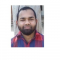## How to remove a column from matrix in R by using its name?Nizamuddin Siddiqui

Updated on 16-Nov-2021 06:23:39

To remove a column from matrix in R by using its name, we can follow the below steps −First of all, create a matrixThen, add names to columns of the matrix.After that, subset the matrix by deselecting the desired column with negation and single square brackets for subsetting.ExampleCreate the matrixLet’s ... Read More

## How to find the row total of columns having same name in data.table object in R?Nizamuddin Siddiqui

Updated on 16-Nov-2021 06:10:07

To find the row total of columns having same name in data.table object in R, we can follow the below steps −First of all, create a data.table with some columns having same name.Then, use tapply along with colnames and sum function to find the row total of columns having same ... Read More

## How to extract the last n values of all elements of a list in R?Nizamuddin Siddiqui

Updated on 16-Nov-2021 06:05:22

To extract the last n values of all elements of a list in R, we can follow the below steps −First of all, create a list.Then, use tail function with sapply function to extract the last n values of all elements in the list.Example 1Create the listLet’s create a list ... Read More

## How to find the row total of columns having same name in R data frame?Nizamuddin Siddiqui

Updated on 16-Nov-2021 05:55:56

To find the row total of columns having same name in R data frame, we can follow the below steps −First of all, create a data frame with some columns having same name.Then, use tapply along with colnames and sum function to find the row total of columns having same ... Read More

## How to apply two sample t test using a categorical column in R data frame?Nizamuddin Siddiqui

Updated on 16-Nov-2021 05:52:55

To apply two sample t test using a categorical column in R data frame, we can follow the below steps −First of all, create a data frame.Then, use t.test function with categorical column and numerical column linked with ~ sign.ExampleCreate the data frameLet’s create a data frame as shown below ... Read More

## How to create a boxplot of single column in R data frame with column name?Nizamuddin Siddiqui

Updated on 16-Nov-2021 05:50:00

To create a boxplot of single column in R data frame with column name, we can follow the below steps −First of all, create a data frame with single numerical column and create the boxplot for that column using boxplot function.Then, create the same boxplot with show.names argument set to ... Read More

## How to find the percentage of values that lie within a range in a single column R matrix?Nizamuddin Siddiqui

Updated on 16-Nov-2021 05:48:18

To find the percentage of values that lie within a range in single column R matrix, we can follow the below steps −First of all, create a matrix.Then, use sum function along with extreme values for range and length function to find the percentage of values that lie within that ... Read More

## How to create a column of product in data frames stored in R list?Nizamuddin Siddiqui

Updated on 16-Nov-2021 05:45:06

To create a column of product in data frames stored in R list, we can follow the below steps −First of all, create a list of data frames.Then, use lapply function to create a column of product in data frames stored in the list.ExampleCreate the list of data framesUsing data.frame ... Read More

## How to combine two rows in R data frame by addition?Nizamuddin Siddiqui

Updated on 16-Nov-2021 05:41:53

To combine two rows in R data frame by addition, we can follow the below steps −First of all, create a data frame.Then, using plus sign (+) to add two rows and store the addition in one of the rows.After that, remove the row that is not required by subsetting ... Read More

## How to replace NAs with 0 in an R list that contains data.table objects?Nizamuddin Siddiqui

Updated on 16-Nov-2021 05:39:54

To replace NAs with 0 in an R list that contains data.table objects, we can follow the below steps −First of all, create a list of data.table objects that contain some NAs.Then, use lapply function and is.na function to replace NAs with 0 in the list.ExampleCreate the listLet’s create a ... Read More

Previous 1 ... 3 4 5 6 7 ... 232 Next